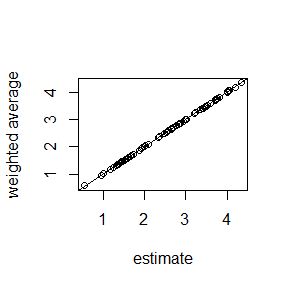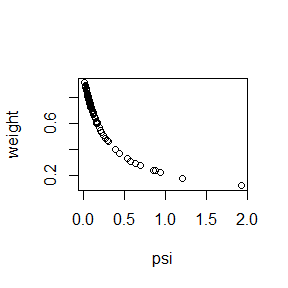# Basic area-level model

The basic area-level model (Fay and Herriot 1979; Rao and Molina 2015) is given by $y_i | \theta_i \stackrel{\mathrm{ind}}{\sim} {\cal N} (\theta_i, \psi_i) \,, \\ \theta_i = \beta' x_i + v_i \,,$ where $$i$$ runs from 1 to $$m$$, the number of areas, $$\beta$$ is a vector of regression coefficients for given covariates $$x_i$$, and $$v_i \stackrel{\mathrm{ind}}{\sim} {\cal N} (0, \sigma_v^2)$$ are independent random area effects. For each area an observation $$y_i$$ is available with given variance $$\psi_i$$.

First we generate some data according to this model:

m <- 75L  # number of areas
df <- data.frame(
area=1:m,      # area indicator
x=runif(m)     # covariate
)
v <- rnorm(m, sd=0.5)    # true area effects
theta <- 1 + 3*df$x + v # quantity of interest psi <- runif(m, 0.5, 2) / sample(1:25, m, replace=TRUE) # given variances df$y <- rnorm(m, theta, sqrt(psi))

A sampler function for a model with a regression component and a random intercept is created by

library(mcmcsae)
model <- y ~ reg(~ 1 + x, name="beta") + gen(factor = ~iid(area), name="v")
sampler <- create_sampler(model, sigma.fixed=TRUE, Q0=1/psi, linpred="fitted", data=df)

The meaning of the arguments used here is as follows:

• the first argument is a formula specifying the response variable and the linear predictor part of the model
• sigma.fixed=TRUE signifies that the observation level variance parameter is fixed at 1. In this case it means that the variances are known and given by psi.
• the function expects precisions rather than variances, and with Q0=1/psi the precisions are set to the vector 1/psi.
• linpred="fitted" indicates that we wish to obtain samples from the posterior distribution for the vector $$\theta$$ of small area means.
• data is the data.frame in which variables used in the model specification are looked up.

An MCMC simulation using this sampler function is then carried out as follows:

sim <- MCMCsim(sampler, store.all=TRUE, verbose=FALSE)

A summary of the results is obtained by

(summ <- summary(sim))
## llh_ :
##       Mean   SD t-value  MCSE q0.05  q0.5 q0.95 n_eff R_hat
## llh_ -28.6 5.86   -4.88 0.123 -38.8 -28.4 -19.7  2285     1
##
## linpred_ :
##    Mean    SD t-value    MCSE q0.05 q0.5 q0.95 n_eff R_hat
## 1  1.70 0.219    7.72 0.00401 1.332 1.69  2.05  3000     1
## 2  4.34 0.314   13.83 0.00646 3.820 4.35  4.86  2361     1
## 3  2.84 0.293    9.71 0.00549 2.366 2.84  3.33  2840     1
## 4  1.96 0.187   10.49 0.00364 1.649 1.96  2.26  2633     1
## 5  2.34 0.148   15.77 0.00271 2.092 2.34  2.58  3000     1
## 6  1.43 0.228    6.26 0.00436 1.049 1.43  1.79  2722     1
## 7  1.33 0.238    5.59 0.00454 0.943 1.33  1.73  2755     1
## 8  1.37 0.378    3.62 0.00689 0.744 1.37  1.98  3000     1
## 9  3.03 0.219   13.83 0.00399 2.660 3.02  3.39  3000     1
## 10 2.77 0.279    9.93 0.00532 2.310 2.78  3.22  2760     1
## ... 65 elements suppressed ...
##
## beta :
##             Mean    SD t-value    MCSE q0.05 q0.5 q0.95 n_eff R_hat
## (Intercept) 1.06 0.145    7.29 0.00767 0.826 1.06  1.30   360  1.01
## x           2.96 0.249   11.88 0.01259 2.536 2.96  3.38   391  1.00
##
## v_sigma :
##          Mean     SD t-value    MCSE q0.05  q0.5 q0.95 n_eff R_hat
## v_sigma 0.508 0.0616    8.24 0.00165 0.414 0.504 0.614  1403     1
##
## v :
##       Mean    SD t-value    MCSE  q0.05    q0.5   q0.95 n_eff R_hat
## 1  -0.4489 0.227  -1.974 0.00572 -0.827 -0.4514 -0.0714  1581 1.001
## 2   0.4102 0.319   1.285 0.00743 -0.125  0.4160  0.9312  1847 1.001
## 3  -0.2350 0.296  -0.795 0.00589 -0.725 -0.2384  0.2594  2523 1.002
## 4  -0.0225 0.202  -0.111 0.00578 -0.361 -0.0179  0.3055  1223 1.003
## 5  -0.6184 0.165  -3.752 0.00458 -0.892 -0.6166 -0.3500  1295 1.002
## 6  -0.4630 0.239  -1.937 0.00602 -0.859 -0.4612 -0.0754  1579 1.001
## 7   0.0312 0.257   0.121 0.00765 -0.384  0.0283  0.4576  1132 1.001
## 8   0.0625 0.380   0.165 0.00780 -0.570  0.0580  0.6936  2367 0.999
## 9   0.4808 0.226   2.129 0.00541  0.107  0.4757  0.8543  1743 1.002
## 10 -0.8638 0.290  -2.978 0.00678 -1.343 -0.8563 -0.4074  1828 1.000
## ... 65 elements suppressed ...

In this example we can compare the model parameter estimates to the ‘true’ parameter values that have been used to generate the data. In the next plots we compare the estimated and ‘true’ random effects, as well as the model estimates and ‘true’ estimands. In the latter plot, the original ‘direct’ estimates are added as red triangles.

plot(v, summ$v[, "Mean"], xlab="true v", ylab="posterior mean"); abline(0, 1) plot(theta, summ$linpred_[, "Mean"], xlab="true theta", ylab="estimated"); abline(0, 1)
points(theta, df$y, col=2, pch=2)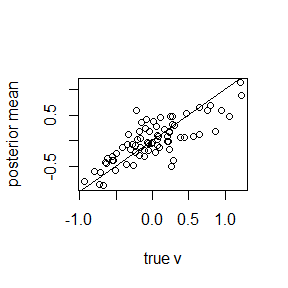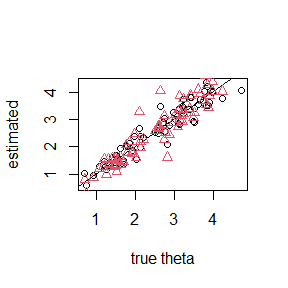We can compute model selection measures DIC and WAIC by compute_DIC(sim) ## DIC p_DIC ## 107.22061 49.99015 compute_WAIC(sim, show.progress=FALSE) ## WAIC1 p_WAIC1 WAIC2 p_WAIC2 ## 78.04790 20.82357 100.73028 32.16476 Posterior means of residuals can be extracted from the simulation output using method residuals. Here is a plot of (posterior means of) residuals against covariate $$x$$: plot(df$x, residuals(sim, mean.only=TRUE), xlab="x", ylab="residual"); abline(h=0)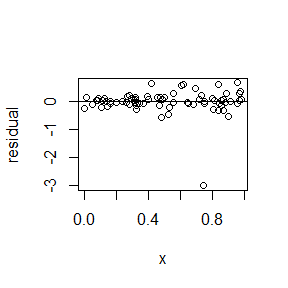A linear predictor in a linear model can be expressed as a weighted sum of the response variable. If we set compute.weights=TRUE then such weights are computed for all linear predictors specified in argument linpred. In this case it means that a set of weights is computed for each area.

sampler <- create_sampler(model, sigma.fixed=TRUE, Q0=1/psi,
linpred="fitted", data=df, compute.weights=TRUE)
sim <- MCMCsim(sampler, store.all=TRUE, verbose=FALSE)

Now the weights method returns a matrix of weights, in this case a 75 $$\times$$ 75 matrix $$w_{ij}$$ holding the weight of direct estimate $$i$$ in linear predictor $$j$$. To verify that the weights applied to the direct estimates yield the model-based estimates we plot them against each other. Also shown is a plot of the weight of the direct estimate for each area in the predictor for that same area, against the variance of the direct estimate.

plot(summ$linpred_[, "Mean"], crossprod(weights(sim), df$y),
xlab="estimate", ylab="weighted average")
abline(0, 1)
plot(psi, diag(weights(sim)), ylab="weight")